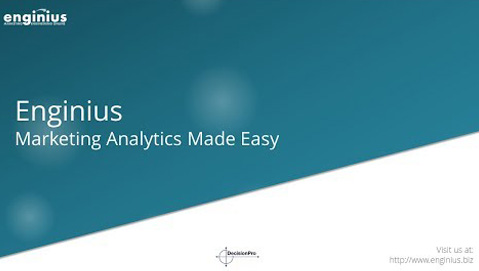• HOME
• REGISTERPlay Video
Help Page

## Predictive modeling

Get answers for the most frequently asked questions about the Enginius predictive modeling module. For a quick overview, we suggest you check the introductory video first.

## Predictive modeling in a nutshellPredictive modeling includes a collection of regression analysis models for estimating the relationships between variables, namely a set of predictors, and one target variable.

The exact type of regression model the module will implement will depend on the kind of target variable, whether it is binary (logit model), categorical (multinomial logit model), continuous (linear regression) or discrete-continuous (logit + linear regression).

First, the regression model is calibrated on a data set where both the predictors and the target variable are known and can be observed.

Once the model has been calibrated, it can be applied to a different data set where only the predictors are observed, to obtain predictions.

Predictive modeling can be used to predict a variety of outcomes, such as discrete probabilities (to purchase, to click, to churn, etc.), likelihood to select a specific brand or to anticipate how much customers will spend.To download the Enginius tutorial in pdf format: (1) Follow the link below. It will open an example data set, then (2) Click on the link in the upper-left corner of the screen.

A discrete-continuous model is the combination of two models: a discrete model that will predict if someone will make a purchase, and a continuous model that will predict the purchase amount (if a purchase occurs). The two models are then combined together to obtain an expected spend amount.

For a discrete-continuous model, the target variable should contain either 0 (no purchase observed) or a positive value representing the amount purchased.

A regression analysis usually assumes that predictors are normally-distributed. If such assumption is violated, it is usually a good idea to transform the predictors using a Box-Cox transformation.

In marketing, many predictors, such as amounts or purchased frequencies, are naturally right-skewed.

For instance, in a typical customer database, many customers will have made one or two purchases at most, whereas only a few will have made a very large number of purchases. Since the first initial purchases contain much more predictive power than later ones (i.e., there is a huge difference between 1 and 2 purchases, but very little between 36 and 37), transforming the predictors might significantly improve the model performance.

You should transform the target variable when it is heavily right-skewed (as you would do with predictors, see above), such as when you try to predict amounts purchased, where many people spend little, and few people spend a lot.

Note that target variables cannot be transformed using a Box-Cox transformation, because the Box-Cox transformation is not guaranteed to be reversible. Only log-transforms are possible for target variables.

Suppose you are trying to predict which customers will make a purchase. If you have 1,000 customers in the data set, and 100 of them have made a purchase, then selecting 250 customers randomly should lead, on average, to 25 observed purchases.

Now, suppose that, instead of selecting 250 individuals randomly, you select the 250 individuals who have the highest likelihood of making a purchase (as predicted by the model). If 90 of these individuals have indeed made a purchase, it means that the model performs significantly better than chance.

In this instance, the model performs (90 / 25) – 1 = 260% better than a random selection. We say that the lift of the model is 3.6. A lift of 1.0 means there is no improvement compared to a random selection. The higher the lift, the better the model